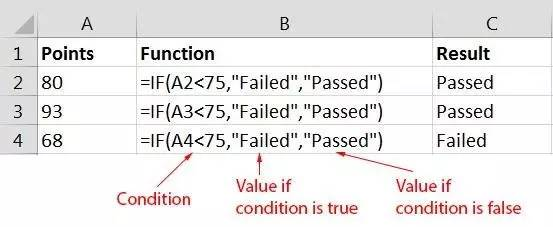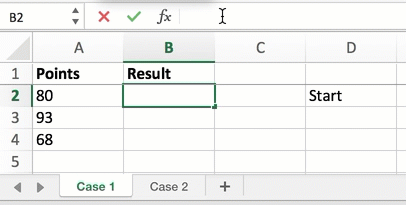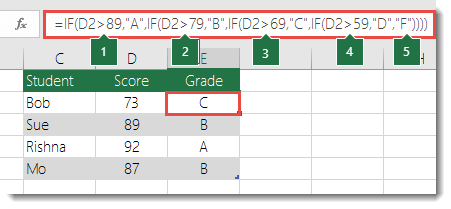Excel 中判断真假的IF 函数的作用介绍及实例应用

Excel 中，IF 函数的作用是根据指定的条件来判断其“真”（TRUE）、“假”（FALSE），从而返回相应的内容。

1IF 函数的语法

IF(logical_test, value_if_true, value_if_false)

2IF 函数实例IF 函数可以叠代使用，从而实现多项条件的判断。IF(D2>89, "A",

IF(D2>89, "A", IF(D2>79, "B",

IF(D2>89, "A", IF(D2>79, "B", IF(D2>69, "C",

IF(D2>89, "A", IF(D2>79, "B", IF(D2>69, "C", IF(D2>59, "D", "F")

IF(D2>89, "A", IF(D2>79, "B", IF(D2>69, "C", IF(D2>59, "D", "F") ) ) )

tag : 真假   IF函数